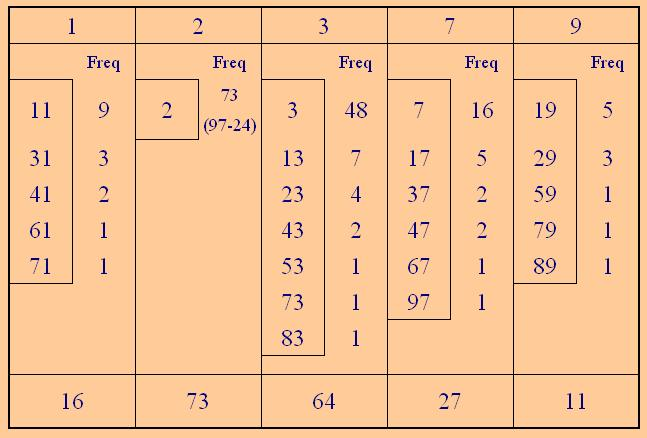#### You may also like### A Biggy

Find the smallest positive integer N such that N/2 is a perfect cube, N/3 is a perfect fifth power and N/5 is a perfect seventh power.### Why 24?

Take any prime number greater than 3 , square it and subtract one. Working on the building blocks will help you to explain what is special about your results.### Factoring a Million

In how many ways can the number 1 000 000 be expressed as the product of three positive integers?

# Fac-finding

##### Age 14 to 16 Challenge Level:

Another well attempted problem with solutions from Mary of Birchwood High School, Sana, Jenny, Chris and Rosion of Madras College, St. Andrews, as well as Andrei of School 205 Bucharest and Chen of the Chinese High School, Singapore.

$$100! = 100 \times99 \times98 \times97 \times97 \times \ldots \times4 \times3 \times2 \times1$$

To write this as the product of its prime factors:

Considering some examples:
$100 = 2^2 \times5^2$
$80 = 2^4 \times5$

So to find all the $2$'s that appear as prime factors it is necessary to consider all the numbers divisible by powers of $2$ between $1$ and $100$
Number of numbers divisible by $2 = 2^1$ is $50$ (the even numbers - with at least one $2$ as a factor)
Number of numbers divisible by $4 = 2^2$ is $25$ (with at least two $2$'s as factors)
Number of numbers divisible by $8 = 2^3$ is $12$ (with at least three $2$'s as factors)
Number of numbers divisible by $16 = 2^4$ is $6$ (with at least four $2$'s as factors)
Number of numbers divisible by $32 = 2^5$ is $3$ (with at least five $2$'s as factors)
Number of numbers divisible by $64 = 2^6$ is $1$(with six $2$'s as factors).

Therefore number of $2$'s is $50 + 25 + 12 + 6 + 3 + 1 = 97$

Similarly:
Number of numbers divisible by $5 = 5^1$ is $20$ (the even numbers - with at least one $2$ as a factor)
Number of numbers divisible by $25 = 5^2$ is $4$ (with at least two $2$'s as factors)

Therefore number of $5$'s is $20 + 4= 24$

Andrei from "Tudor Vianu" National College, Bucharest, confirmed that $100!$ Has $24$ zeros are at the end of this large number. He then provided an excellent table which he could then use to see that the first digit to precede them was $4$Here's how this table works. There are a lot of prime factors in $100!$ but Andrei has organised the data so that counting them isn't too hard.

We already know there are $97$ prime factors that are $2$ ($24$ of which are used with the available fives to create those $24$ trailing zeros)

$100!$ means one multiplied with two multiplied with three . . . . multiplied with $100$.

Which of all those factors will contain a prime factor of three?

$3, 6, 9,\ldots 99$ all will (that's $33$ so far)

Which will contain a second prime factor of three?

$9, 18, 27 \ldots99$ (that's $11$ more, so now $44$ so far)

which will contain a third prime factor of three?

$27, 54, 8$1 (that's $3$ extra) and $81$ has four prime factors of $3$ (so one more, making $48$ in all)

Find that "$48$", showing the frequency of the prime factor of three, in Andrei's table.

The fives have all been used up getting the zeros.

There's less work involved in doing the sevens:

$7, 14, 21,$ to $98$ ( $14$ so far )

$49$ and $98$ have a second factor of $7$ (total $16$, find it in the table)

There are only $25$ prime numbers smaller than one hundred so checking isn't too big a job, and the bigger numbers involve almost no work so don't be discouraged.

Now the smart thing about Andrei's table is the way the numbers are arranged.

It's the final (units) digit that we are interested in so it's only the last digit we need to consider. For example the prime factors of $11, 31, 41, 61,$ and $71$ will all have the same effect on that last digit. Look down the other columns in the table to see similar groupings and the frequency total at the bottom.

Now all the ones will create a final one.

Numbers ending in $3$ will form a product whose final digit will depend on how many numbers were multiplied but will follow a cycle of $3, 9, 7, 1$ then $3$ again. So with $64$ numbers multiplied the final digit is a one.

Reasoning likewise the numbers ending in $7$ have a product ending in $3$, and the $11$ prime factors ending in $9$ will produce a final digit of $9$

The twos follow a cycle and the last digit of their product is $2$.

Finally the product of all those last digits has a last digit of $4$, so $4$ is the digit that immediately precedes the zeros.

Many thanks Andrei.